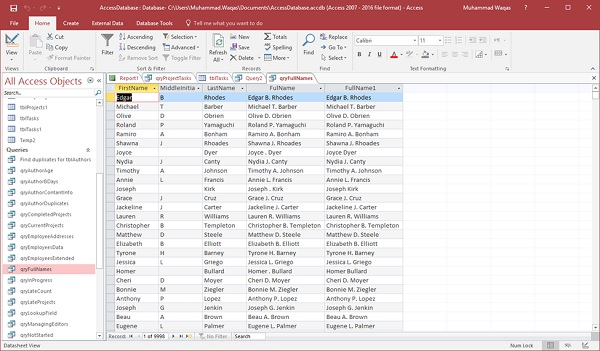# MS Access

In this chapter, we will be working with Built-in Functions. In Access, there are close to a hundred built-in functions and it is almost impossible to cover every single one of them. In this chapter, we will cover the basic structure, syntax, and use some of the more popular functions, and also the pitfalls, so that you can go exploring some of the other functions on your own.

## Functions

A function is a VBA procedure that performs a task, or calculation, and returns a result. Functions can generally be used in queries, but there are other places that you can use functions.

• You can use functions in table properties, for example, if you want to specify a default value for a date/time field, you can use the date or the Now function to call up the current date/time information from your system, and input that value automatically.

• You can also use functions in an expression when you create a calculated field, or use the functions inside form or report controls. You can use functions even in macro arguments.

• Functions can be quite simple, requiring no other information to be called, or, simply reference one field from a table or query.

• On the other hand, they can also get quite complicated, with multiple arguments, field references, and even other functions nested inside another function.

Let us now look into some examples of using built-in functions.

## Date & Time Functions

Let us now understand the Date and Time functions −

• The Date() function is designed to return the current system date. This function does not require any function arguments or additional information. All you have to do is write the name of the function and those open and close parentheses.

• There are two very similar built-in functions Time() and Now().

• The Time() Function returns the current system time only and the Now() Function returns both the current system date and time.

• Depending on the data that you want to track, or store, or query, you have three built-in, easy-to-use functions to help with that task.

Let us now open your database and create a new query using query design and add tblProjects and tblTasks.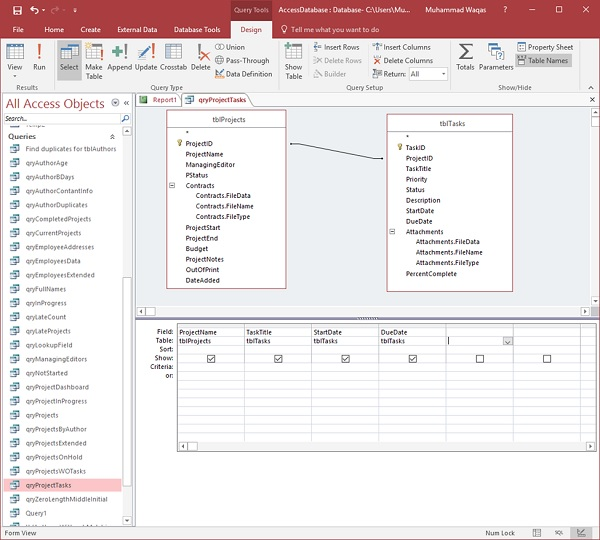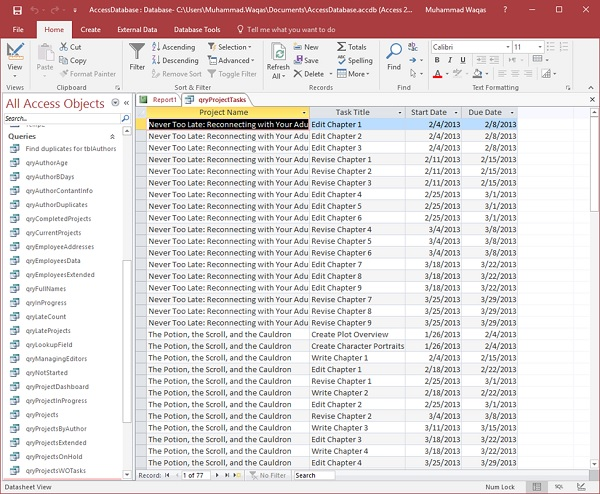You can now see all the different tasks from all projects. If you want to view the project tasks that are in progress as on today’s date, then we have to specify a criterion using a Date() Function to look at projects that start on or after today’s date.

Let us now specify the criteria underneath the StartDate.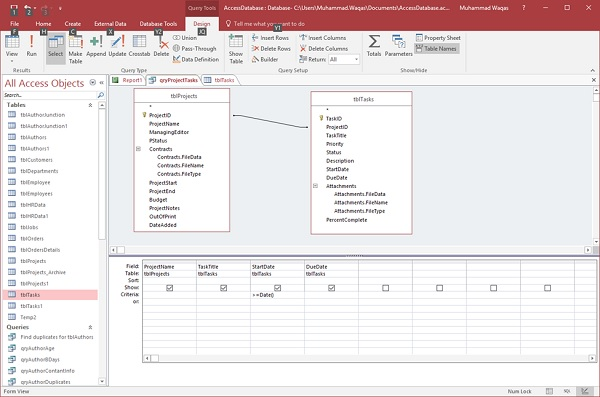The criteria starts with an operator greater than symbol, followed by an equal to symbol and then Date Function.

When we run this query, all the tasks will occur either on today’s date or in the future as in the following screenshot.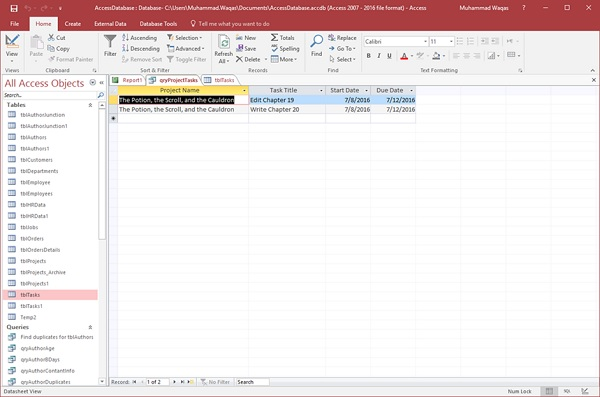This was an example of how you can use the Date() function as query criteria.

• Let us now say this query needs to be more flexible in terms of the dates it is pulling starting this week.

• We do have a couple of different tasks that began this week, that are not showing up in this current list, because of our criteria. It’s looking at start dates that are equal to today or above.

If we want to view the tasks that started this week, that have not yet completed or should complete today, let us go back to the Design View.

Here, we will add some additional information to these criteria. In fact, we want it greater than or equal to today’s date minus seven days.If we type minus seven and run the query, you can see the tasks that started this week as well.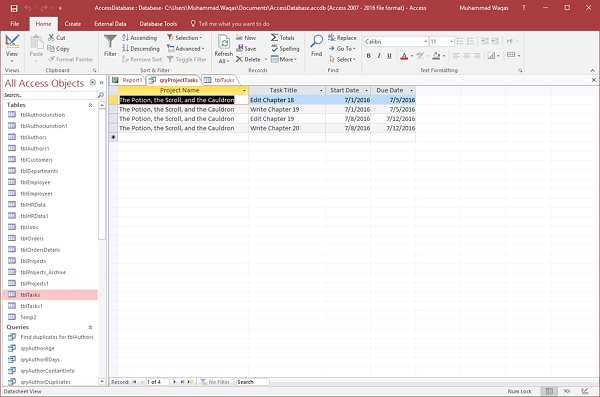## DateDiff() Function

The DateDiff() Function is another very popular date/time function. The DateDiff Function returns a Variant (long), specifying the number of time intervals between two specified dates. In other words, it calculates the difference between two dates, and you get to pick the interval by which the function calculates that difference.

Let us now say we want to calculate our authors’ age. For this, we first we need to create a new query and add our authors table and then add FirstName, LastName, and the BirthDay fields.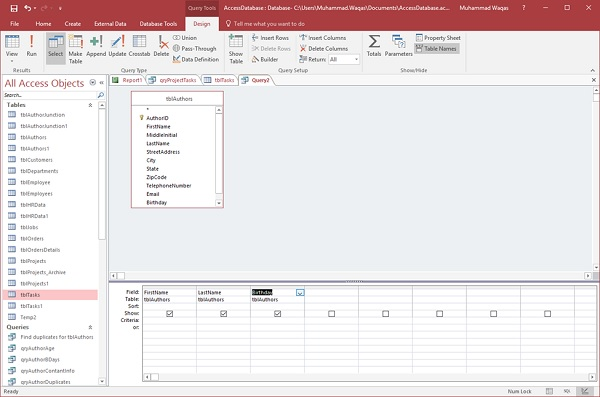We can calculate people’s age by calculating the difference between their date of birth, or birthday and whatever today’s date is.

Let us try using the DateDiff Function in a new field.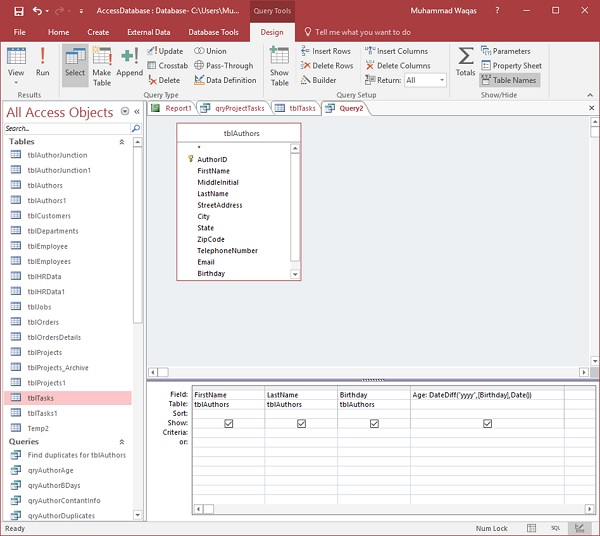Let us call it Age followed by a colon, and then write DateDiff Function.

• The first function argument for the DateDiff function is the interval, so type “yyyy”.
• The next function argument is the first date that we want to calculate by, which, in this case, will be the Birthday field.
• The third function argument is whatever today’s date is.

Now, run your query and you will see the new field which shows the age of each author.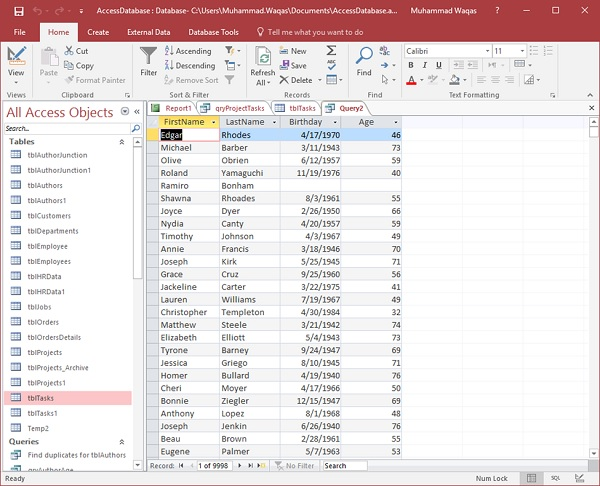## Format() Function

The Format() Function returns a string, containing an expression formatted according to instructions contained in a format expression. Here is the list of user-defined formats which can be used in Format() function.ss

Setting Description
yyyy Year
q Quarter
m Month
y Day of year
d Day
w Weekday
ww Week
h Hour
n Minute
s Second

Let us now go back to your query and add more fields in the same using the Format() function.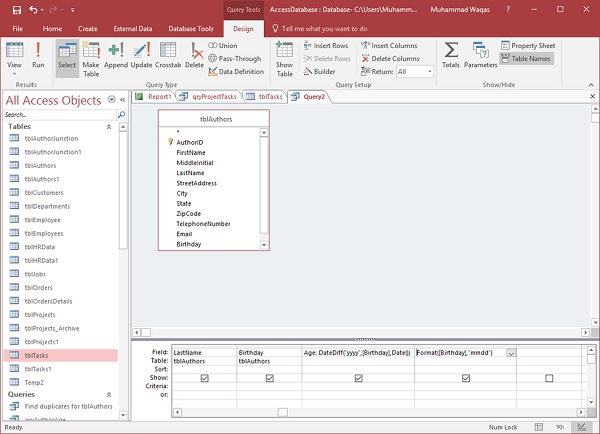Type the Format Function. The first function argument will be an expression, which can be almost anything. Let us now have the birthday field as the first and the next thing is to write our format. In this case, we need month, month, day, day. Write “mmdd” in quotes and then, run your query.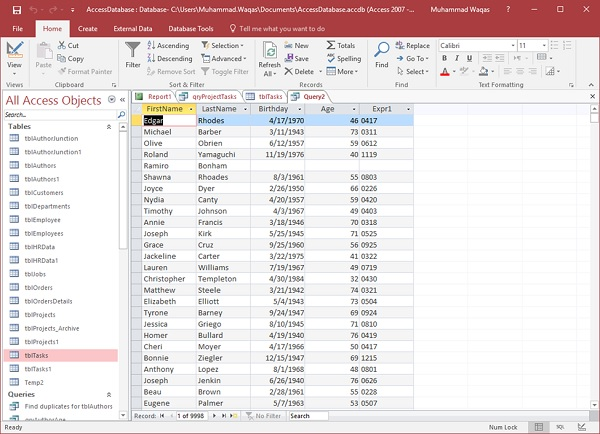It is now taking the date from the birthday field, 4 is the month and 17 is the day.

Let us add “mmm” and “mmmm” instead of “mmdd” in the next fields as in the following screenshot.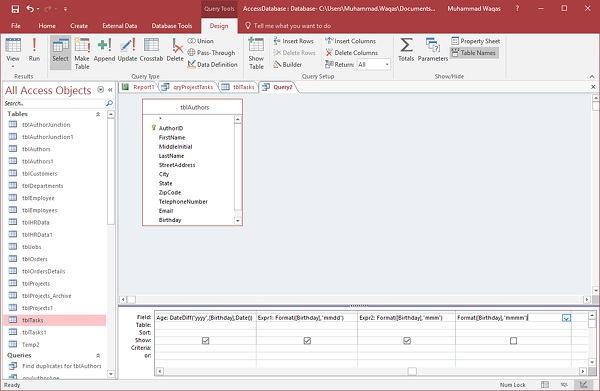Run your query and you will see the results as in the following screenshot.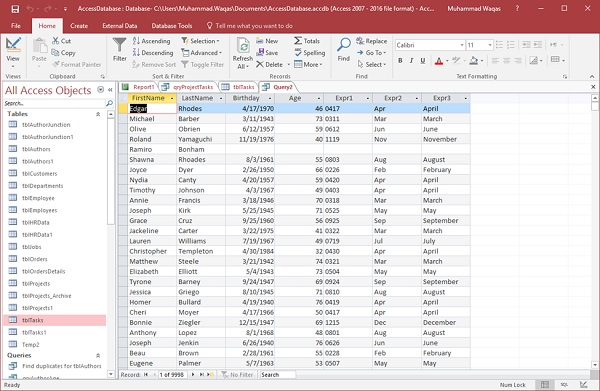In the next field, it is returning the first 3 character from the name of the month for that birthday and in the last field you will get the full month name.

To see the month followed by year from birthday, let us add the “yyyy” as well as shown in the following screenshot.Let us run your query again.You will now see the month followed by a comma and then the year.

## IIf() Function

The IIf() Function is an abbreviation for “Immediate If” and this function evaluates an expression as either true or false and returns a value for each. It has up to three function arguments, all of which are required.

• The first argument is any expression that you want to evaluate.
• The next argument stands for the true part, which can be a value or an expression returned if your first expression is true.
• The last argument is what you want returned if your expression is false.

### Example

Let us take a simple example. We will create a new query using query design and add tblAuthors table and then add the following fields.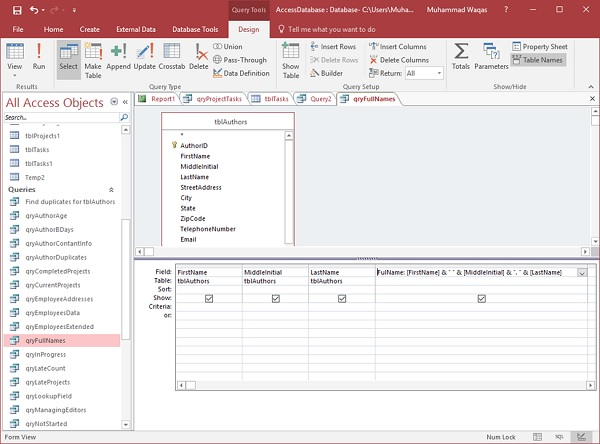You can now see we have three fields — FirstName, MiddleInitial, LastName, and then this concatenated field, which is pulling all three fields together. Let us run your query to see the result of this query.Now, you can see the result of the query, but you will also notice that some records do not have a middle initial. For example, the Joyce Dyer record does not have a middle initial, but in the FullName field you will see the period that really doesn’t need to be there. So, go back to the Design View. Here, we will concatenate the name in a different way using the IIf Function.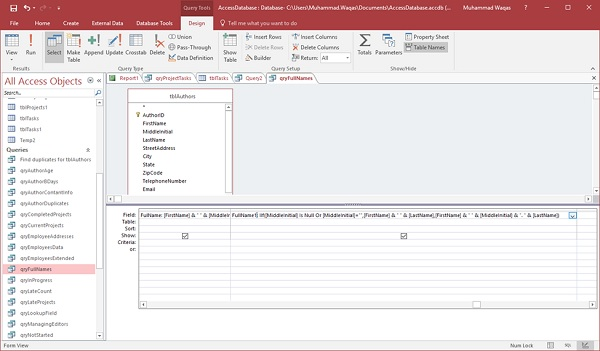Let us write the name in another field and call it FullName1 and then type the IIf function.

• The first function argument for the Immediate If function is going to be your expression. In the expression, we will see if the middle initial field is blank or is null.

• The next argument is the true part. So, if the middle initial is null then we would want to display the FirstName and the LastName.

• Now, for our false part — if the MiddleInitial is not null, then we would want to display the FirstName, MiddleInitial, and LastName.

Let us now run your query and you will see the results as in the following screenshot.